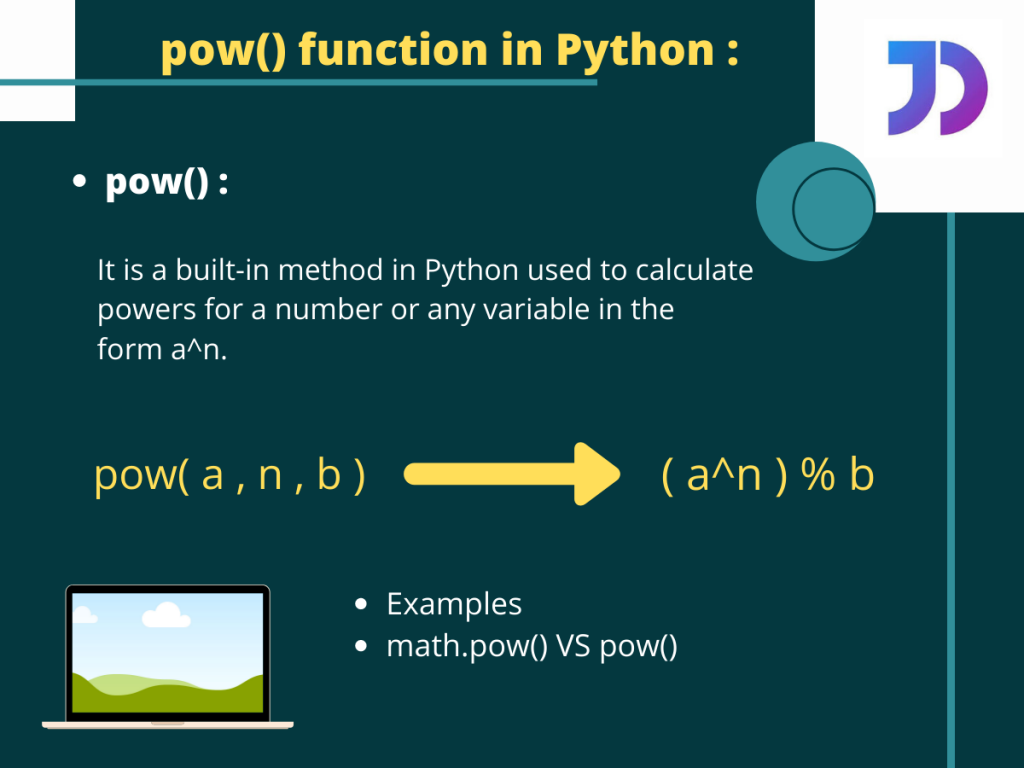# Python pow() methodThe Python `pow()` function is one of the most commonly used built-in function in Python programming. It is extensively used to calculate the value of a to the power n or more specifically an. It is a very useful function when dealing with some complex mathematical calculations or sometimes for other operations. So, let us dig a bit deeper into the `pow()` function in Python.

## Using the Python pow() function

The `pow()` function can be passed with a total of three arguments. The syntax for `pow()` is given below,

`pow( a , n , b )`

Where,

• a is the number whose power we are calculating or the base number,
• n is how much to the power a is to be raised or the exponential part,
• b is the number with which the modulus of an is going to be calculated.

Note: b is an optional argument.

## Examples

Look at the code below, we here try to calculate the value of, let us say, 25.

```x=pow(2,5)     #2^5
y=pow(4,-2)    #1/(4^2)
print(x,y)
```

Output:

Lets again try passing the optional modulus argument now,

```x=pow(2,5,5)        #(2^5) % 5 = 32 % 5
print(x)
```

Output:

```2
```

So accordingly we get the output as 2. Since `pow(2,5,5)` actually returns the value for (2^5) % 5 Or, 32 % 5 = 2.

Note: While using the modulo argument we must make sure that the 2nd argument(exponent part) is a positive integer. Or else an error is thrown as shown below,

```y=pow(4,-2,6)
print(y)
```

Output:

```Traceback (most recent call last):
File "C:/Users/sneha/Desktop/test.py", line 2, in <module>
y=pow(4,-2,6)
ValueError: pow() 2nd argument cannot be negative when 3rd argument specified
```

## math.pow() vs. Built-in pow() in Python

Apart from the fact that the `math.pow()` doesn’t come with the integrated modulus operation, both the built-in `pow()` and the pre-defined `math.pow()` have some big differences.

The `pow()` function is comparatively faster for large set of values. On the other hand for using math.pow() the user has to first import math module.

Even math.pow() method throws an error while calculating the results for some complex numbers. Which the pow() method does not.